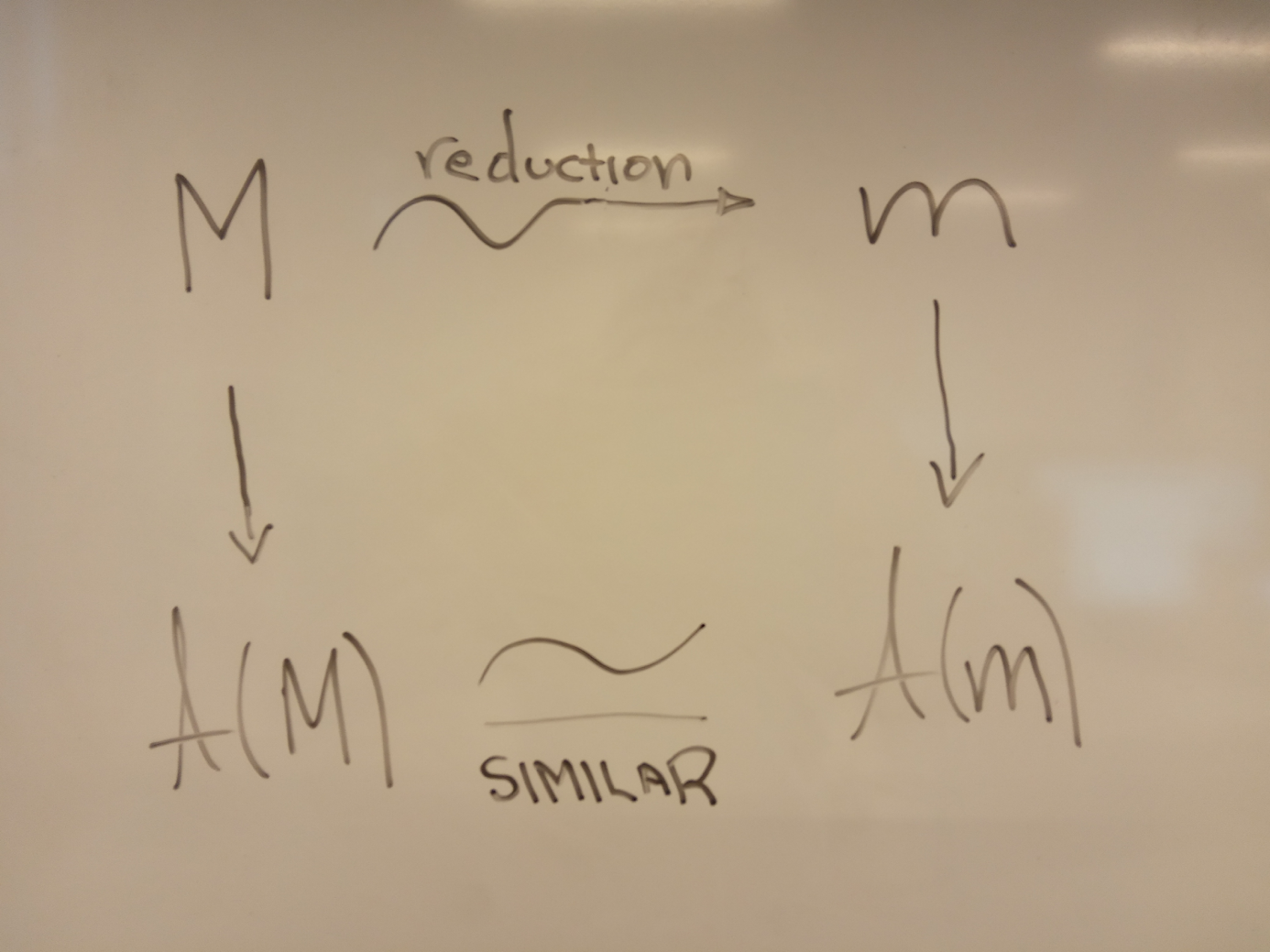# A Novel Approach to the Reduction of Boolean Networks

Georgios Argyris: Simplifying complex systems

Mathematical models aim at an effective representation of real-world complex systems.  A high dimensional mathematical model can precisely describe a system as it inherits its complexity and consequently retains its interpretability. However, high dimensionality and computational restrictions render model analysis, interpretation and inference, a hard task. Hence the modeller needs to find the trade-off between precision and tractability. In the context of dynamical models several reduction techniques have been proposed that diminish dimensionality while maintaining the system’s properties of interest.

Stochastic processes, differential equations, transitions systems and automata have been some of the most common forms of mathematical modelling. Although, network science has also provided useful tools for simulating real-world complex systems. Specifically, Boolean Networks introduced by Kaffman in 1969 have arisen as ideal candidates for modeling biological functions like gene regulation, brain function etc. A Boolean Network (BN) is a discrete time dynamical model which consists of nodes and links. The number of nodes represents the dimensionality of the model as they denote the variables/species of the system. Each node owns values (0 or 1 in the default case) and update functions whereas links represent the influences/interactions between nodes.

BN dynamics (the state space) are encoded into state transition graphs (STGs). Each node of an STG is a particular instance/configuration of the BN whereas the directed edges denote the transition at a time step. Several emergent BN properties are identified in STGs like attractors and their basins. Attractor is a set of states towards which a system tends to evolve and remain whereas an attractor’s basin of attraction is a set of states such that any state in the set will eventually guide the system into the attractor. These properties are usually associated with the interpretation of the underlying system e.g. different attractors correspond to different types of cells during cell differentiation. Unfortunately, as the dimensionality of a Boolean network increases, the corresponding STG grows exponentially, a fact that hampers the analysis of the BN and consequently the study of the underlying system.

In this project we are going to introduce a novel mathematical framework for the reduction (denoted with arrow in the figure below) of a Boolean network M into a smaller one m preserving its properties of interest stated above and providing good description of the original system with interpretability and low-cost but similar analysis (denoted with A in figure below). We plan to introduce methods that will rely on the notions of bisimulation and satisfiability of Boolean formulas and will be accompanied by software implementations offering usable and efficient tool support and facilitating BN analysis to interdisciplinary researchers.

## PhD project

By: Georgios Argyris

Section: Software Systems Engineering

Principal supervisor: Alberto Lluch Lafuente

Co-supervisor: Andrea Vandin

Term: 01/02/2020 → 31/01/2023

## Contact## ContactAlberto Lluch Lafuente